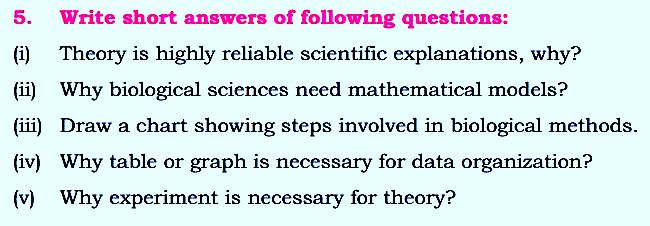# Biology Class 9 Notes Chapter 2

## Biology Class 9 Notes Chapter 2Biology Class 9 Notes

Chapter 2 of Biology Class 9 typically covers the topic of "Cell Structure and Function". This chapter introduces the basic unit of life, the cell, and discusses its various parts and functions.

### The theory is a highly reliable scientific explanation, why?

The theory is a highly reliable scientific explanation because it is based on empirical evidence collected through observation, experimentation, and data analysis. Theories are developed and tested over time and only become widely accepted if they consistently explain and predict phenomena.

### Why do biological sciences need mathematical models?

Biological sciences need mathematical models because biology is a complex and dynamic field that involves many different processes and interactions. Mathematical models provide a way to represent and analyze these processes and interactions, allowing scientists to make predictions and test their theories.

### Draw a chart appearing the steps included in natural methods.

The steps involved in biological methods include: observing and defining the problem, formulating a hypothesis, designing and conducting experiments, collecting and analyzing data, interpreting the results, and drawing conclusions.

### Why table or graph is important and necessary for data organization?

A table or graph is necessary for data organization because it provides a clear and concise way to present and summarize large amounts of information. Tables and graphs help to identify trends, patterns, and relationships in the data, making it easier to analyze and interpret the results.

### Why experiment is necessary for theory?

Experiment is necessary for theory because theories are developed through a process of experimentation and observation. Experiments are used to test and validate theories, and the results of experiments can be used to refine or reject theories. Without experiments, theories would remain unproven and unreliable.